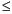Functions and CALL Routines

# PERM Function

Computes the number of permutations of n items that are taken r at a time.
 Category: Combinatorial

## Syntax

 PERM(n<,r>)

### Arguments

n

is an integer that represents the total number of elements from which the sample is chosen.

r

is an integer value that represents the number of chosen elements. If r is omitted, the function returns the factorial of n.

 Restriction: rn

The mathematical representation of the PERM function is given by the following equation:with n0, r0, and nr.

If the expression cannot be computed, a missing value is returned. For moderately large values, it is sometimes not possible to compute the PERM function.

SAS Statements Results
`x=perm(5,1);`
`5`
`x=perm(5);`
`120`
`x=perm(5,2)`
`20`

 Functions:Previous Page | Next Page | Top of Page